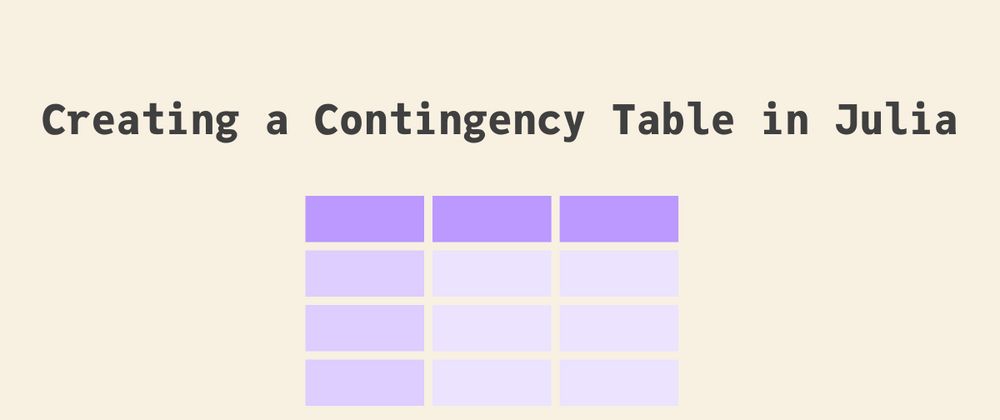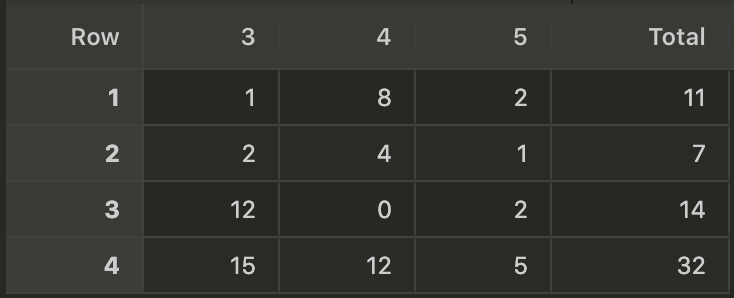## Julia Community 🟣Alex Tantos

Posted on • Updated on

# Creating a Contingency Table in Julia

## Why are contingency tables useful?

More often than not, our data sets include categorical variables that encode qualitative features of our data and take a limited number of possible discrete values. A very common scenario is to check whether there is an association between pairs of such variables. For instance, to check whether the type of car is associated with the number of gears or the number of cylinders in the well-known `mtcars` dataset included in the `RDatasets` package.1

There are numerous measures invented especially for measuring whether pairs of categorical variables are associated and used in scientific fields such as linguistics, biology and physics.

## What is a contingency table?

However, to be able to calculate such association measures between pairs of categorical variables, it is essential to prepare a contingency table.

A contingency table is a two-dimensional table whereby each of the rows represents one level of the first categorical variable and each of the columns represents one level of the second categorical variable.

To be able to work efficiently with this type of data, you first need to assign these columns the right type that maps to the statistically-meant data type and retains the information about the levels of the categorical variable and any ordering that they may have. In other words, you need to find a data type that corresponds to factors in `R`. A common -but not unique- way to represent categorical data in `Julia` is through the `CategoricalArray` data type.2

Let's create the contingency table of the number of gears and the number of cylinders. Both of these variables are interpreted as `Integer`s, but their values are discrete and, clearly, categorical in nature.

``````using DataFrames, Chain, RDatasets, FreqTables

cars = dataset("datasets", "mtcars")

``````

## Extracting and converting the two categorical variables to `CategoricalArray`s

Although the two variables are read/parsed as `Integer`s, they should be first converted to `CategoricalValue`s. The following code first converts the `Integer` to `String` values with the `string()` function and then to `CategoricalValues` with the `CategoricalArray()` constructor.

``````cars[!,[:Gear, :Cyl]] = @chain cars begin
combine(_, [:Gear, :Cyl] .=> x -> CategoricalArray(string.(x)), renamecols=false)
end
``````

## Creating the contingency table

``````cyl_gear_freq = freqtable(cars, :Cyl, :Gear)
``````

To be able to add the column with the row totals, you need to apply the relevant transformation that a) takes a tuple of the column names with the `AsTable()` function, b) applies the sum function to it and c) names the column `Total`.

Notice that `cyl_gear_freq` is of type `NamedMatrix` and in order to transform it to a `DataFrame`, we need to get the array of its values, you would need to use the `array` field. Moreover, since its a two-dimensional object, it includes two axes of names and we need the second one so that we can assign names to the new `DataFrame`.

``````cyl_gear = @chain DataFrame(cyl_gear_freq.array, Symbol.(names(cyl_gear_freq))) begin
transform!(AsTable(All()) => sum => :Total)
end
``````

Finally, to add the column total, you can use `push!()` that adds the resulting array, created by a comprehension with the column totals, to `cyl_gear`.

``````push!(cyl_gear, [sum(col) for col in eachcol(cyl_gear)])
``````

## The final result

Here is how the contingency table with its margins looks like:1. Recall that the `RDatasets` imports the pool of commonly used dataset when `R` is loaded.

2. This data type is also recommended by the `DataFrames.jl` package documentation as well as the recently-published book Julia for Data Analysis, written by Bogumil Kaminski, for expressing categorical variables in `Julia`